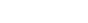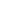xElastic modulusEncyclopedia
An elastic modulus, or modulus of elasticity, is the mathematical description of an object or substance's tendency to be deformed elastically (i.e., non-permanently) when a force
Force
In physics, a force is any influence that causes an object to undergo a change in speed, a change in direction, or a change in shape. In other words, a force is that which can cause an object with mass to change its velocity , i.e., to accelerate, or which can cause a flexible object to deform...

is applied to it. The elastic modulus of an object is defined as the slope
Slope
In mathematics, the slope or gradient of a line describes its steepness, incline, or grade. A higher slope value indicates a steeper incline....

of its stress–strain curve in the elastic deformation region: As such, a stiffer material will have a higher elastic modulus.where lambda (λ) is the elastic modulus; stress
Stress (physics)
In continuum mechanics, stress is a measure of the internal forces acting within a deformable body. Quantitatively, it is a measure of the average force per unit area of a surface within the body on which internal forces act. These internal forces are a reaction to external forces applied on the body...

is the restoring force caused due to the deformation divided by the area to which the force is applied; and strain
Strain (materials science)
In continuum mechanics, the infinitesimal strain theory, sometimes called small deformation theory, small displacement theory, or small displacement-gradient theory, deals with infinitesimal deformations of a continuum body...

is the ratio of the change caused by the stress to the original state of the object. If stress is measured in pascal
Pascal (unit)
The pascal is the SI derived unit of pressure, internal pressure, stress, Young's modulus and tensile strength, named after the French mathematician, physicist, inventor, writer, and philosopher Blaise Pascal. It is a measure of force per unit area, defined as one newton per square metre...

s, since strain is a dimensionless quantity, then the units of λ are pascals as well.

Since the denominator becomes unity if length is doubled, the elastic modulus becomes the stress induced in the material,when the sample of the material turns double of its original length on applying external force. While this endpoint is not realistic because most materials will fail before reaching it, it is practical, in that small fractions of the defining load will operate in exactly the same ratio. Thus, for steel with a Young's modulus
Young's modulus
Young's modulus is a measure of the stiffness of an elastic material and is a quantity used to characterize materials. It is defined as the ratio of the uniaxial stress over the uniaxial strain in the range of stress in which Hooke's Law holds. In solid mechanics, the slope of the stress-strain...

of 30 million psi, a 30 thousand psi load will elongate a 1 inch bar by one thousandth of an inch; similarly, for metric units, where a thousandth of the modulus in gigapascals will change a meter by a millimeter.

Specifying how stress and strain are to be measured, including directions, allows for many types of elastic moduli to be defined.
The three primary ones are:
• Young's modulus
Young's modulus
Young's modulus is a measure of the stiffness of an elastic material and is a quantity used to characterize materials. It is defined as the ratio of the uniaxial stress over the uniaxial strain in the range of stress in which Hooke's Law holds. In solid mechanics, the slope of the stress-strain...

(E) describes tensile elasticity
Elasticity (physics)
In physics, elasticity is the physical property of a material that returns to its original shape after the stress that made it deform or distort is removed. The relative amount of deformation is called the strain....

, or the tendency of an object to deform along an axis when opposing forces are applied along that axis; it is defined as the ratio of tensile stress to tensile strain. It is often referred to simply as the elastic modulus.
• The shear modulus or modulus of rigidity (G or) describes an object's tendency to shear (the deformation of shape at constant volume) when acted upon by opposing forces; it is defined as shear stress
Shear stress
A shear stress, denoted \tau\, , is defined as the component of stress coplanar with a material cross section. Shear stress arises from the force vector component parallel to the cross section...

over shear strain. The shear modulus is part of the derivation of viscosity
Viscosity
Viscosity is a measure of the resistance of a fluid which is being deformed by either shear or tensile stress. In everyday terms , viscosity is "thickness" or "internal friction". Thus, water is "thin", having a lower viscosity, while honey is "thick", having a higher viscosity...

.
• The bulk modulus
Bulk modulus
The bulk modulus of a substance measures the substance's resistance to uniform compression. It is defined as the pressure increase needed to decrease the volume by a factor of 1/e...

(K) describes volumetric elasticity, or the tendency of an object to deform in all directions when uniformly loaded in all directions; it is defined as volumetric stress over volumetric strain, and is the inverse of compressibility. The bulk modulus is an extension of Young's modulus to three dimensions.

Three other elastic moduli are Poisson's ratio
Poisson's ratio
Poisson's ratio , named after Siméon Poisson, is the ratio, when a sample object is stretched, of the contraction or transverse strain , to the extension or axial strain ....

, Lamé's first parameter, and P-wave modulus
P-wave modulus
In linear elasticity, the P-wave modulus M, also known as the longitudinal modulus, is one of the elastic moduli available to describe isotropic homogeneous materials....

.

Homogeneous and isotropic (similar in all directions) materials (solids) have their (linear) elastic properties fully described by two elastic moduli, and one may choose any pair. Given a pair of elastic moduli, all other elastic moduli can be calculated according to formulas in the table below at the end of page.

Inviscid fluids are special in that they cannot support shear stress, meaning that the shear modulus is always zero. This also implies that Young's modulus is always zero.

• Bending stiffness
Bending stiffness
The bending stiffness E I of a beam relates the applied bending moment to the resulting deflection of the beam. It is the product of the elastic modulus E of the beam material and the area moment of inertia I of the beam cross-section...

• Dynamic modulus
Dynamic modulus
Dynamic modulus is the ratio of stress to strain under vibratory conditions...

• Elastic limit
• Elastic wave
• Flexural modulus
Flexural modulus
In mechanics, the flexural modulus is the ratio of stress to strain in flexural deformation, or the tendency for a material to bend. It is determined from the slope of a stress-strain curve produced by a flexural test , and uses units of force per area...

• Hooke's Law
Hooke's law
In mechanics, and physics, Hooke's law of elasticity is an approximation that states that the extension of a spring is in direct proportion with the load applied to it. Many materials obey this law as long as the load does not exceed the material's elastic limit. Materials for which Hooke's law...

• Impulse excitation technique
Impulse excitation technique
-Practical:The impulse excitation technique is a nondestructive test method that uses natural frequency, dimensions and mass of a test-piece to determine Young's modulus, Shear modulus, Poisson's ratio and damping coefficient....

• Proportional limit
• Stiffness
Stiffness
Stiffness is the resistance of an elastic body to deformation by an applied force along a given degree of freedom when a set of loading points and boundary conditions are prescribed on the elastic body.-Calculations:...

• Tensile strength
Tensile strength
Ultimate tensile strength , often shortened to tensile strength or ultimate strength, is the maximum stress that a material can withstand while being stretched or pulled before necking, which is when the specimen's cross-section starts to significantly contract...

• Transverse isotropy
Transverse isotropy
A transversely isotropic material is one with physical properties which are symmetric about an axis that is normal to a plane of isotropy. This transverse plane has infinite planes of symmetry and thus, within this plane, the material properties are same in all directions...